Difference set

(diff) ← Older revision | Latest revision (diff) | Newer revision → (diff)

complete difference set

A setconsisting ofresidues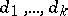modulo a certain natural numbersuch that for each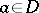,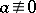(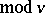), there exist preciselyordered pairs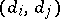of elements fromfor which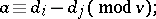the numbers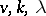are called the parameters of the difference set. For example, the set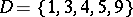of residues modulo 11 is a difference set with.

Difference sets are closely connected with block designs (cf. Block design), namely: The existence of a difference set is equivalent to the existence of a symmetric block design with parameters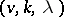having a cyclic group of automorphisms of order(the blocks of such a design are the sets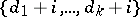,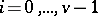). The notion of a difference set can be generalized in the following way: A setconsisting ofdistinct elementsof a groupof orderis called a-difference set inif for any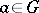,, there exist preciselyordered pairs,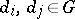, such that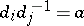(or, what is the same,pairswith). In this case a difference set as defined above is called a cyclic difference set (since the group of residue classesis a cyclic group). The existence of-difference sets in a groupof orderis equivalent to the existence of a symmetric block design with parametersadmittingas a regular (that is, without fixed points) group of automorphisms (this design is obtained by identifying the elements of the block design with the elements of the group and the blocks with the sets, whereruns over).

The question of the existence and construction of a difference set with given parameters is fundamental in the theory of difference sets. For this question the concept of a multiplier of a difference set turns out to be useful: An automorphism of the groupis a multiplier of a-difference setinif it is also an automorphism of the block design determined by. For a cyclic difference set a multiplier is a numberrelatively prime withand with the property thatfor a certain,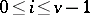. The multipliers of a cyclic difference set form a group. The following assertion is true: Ifis a cyclic-difference set and ifis a prime number dividing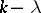and such thatand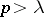, thenis a multiplier of(the multiplier theorem for difference sets). The following result is useful when constructing difference sets: For any multiplier of a-difference setin an Abelian groupof orderthere exists a block in the block design determined bywhich is fixed by this multiplier; when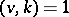there exists a block fixed by any multiplier.

Difference sets are usually constructed by direct methods, using the properties of finite fields and cyclotomic fields (see Cyclotomic field) as well as finite geometries. Several infinite families of difference sets are known, for example the following typesand.

Type(Singer difference sets): These are hyperplanes in an-dimensional projective geometry over a field ofelements. The parameters are: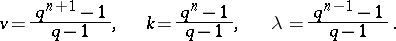Type: Quadratic residues in the field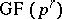when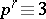(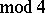) (is a prime number). The parameters are:For other infinite families of difference sets see . Besides these difference sets, generalized difference sets are often considered. They are also called difference families and are sets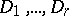consisting of residuessuch that for any() there exist preciselyordered pairs,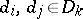, with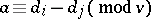for a certain,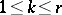.

There are also other generalizations of difference sets.

How to Cite This Entry:
Difference set. Encyclopedia of Mathematics. URL: http://encyclopediaofmath.org/index.php?title=Difference_set&oldid=14897
This article was adapted from an original article by V.E. Tarakanov (originator), which appeared in Encyclopedia of Mathematics - ISBN 1402006098. See original article